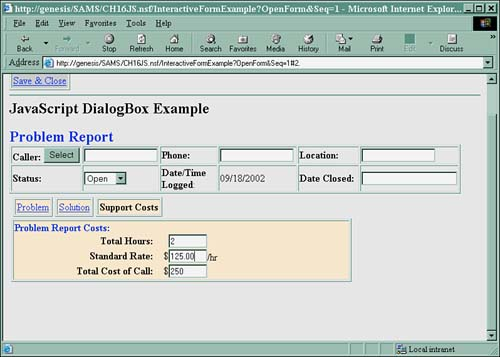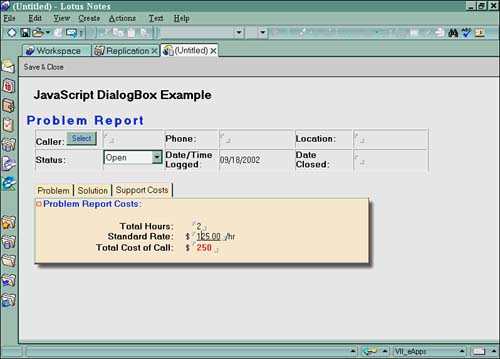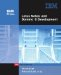# Calculations

JavaScript enables on-the-fly calculations to be scripted for use in Notes as well as on the Web. If you have an application that will be used in both Notes and the Web, JavaScript allows you to code one solution for both. Here's how.

This example takes you through some simple math. The first thing you have to keep in mind is that all fields in JavaScript are evaluated literally. If the number field format is set to currency, the currency sign will be included in the calculation and will result in a NaN. In this example, a calccosts () function has been created in the JS Header on the problem report form in the sample database. Here's the code:

```function calccosts() {
myform = document.forms;
var result = eval(myform.Hours.value * myform.Rate.value);
myform.TotalCost.value = result;
}
```

This function multiplies the two fields Hours and Rate on the form and sets the var result with the result. The TotalCost field is then set with the value of the var result . The Hours, Rate, and TotalCost field formats are all set to a number, decimal, or varying data type on the form.

To call the function, place the calccosts() statement in the onBlur event of the Hours and Rate fields. When either field is exited, the calccosts() function is called. Figure 16.20 shows how it looks in Domino Designer.

Figure 16.20. Calling the calccosts() function in Domino Designer.Figure 16.21 shows how the result looks on the Web.

Figure 16.21. The calculation code results on the Web.Figure 16.22 shows how the same result looks in Notes.

Figure 16.22. The calculation code results in Notes.The differences are only in the client ”the JavaScript code works the same in both.Lotus Notes and Domino 6 Development (2nd Edition)
ISBN: 0672325020
EAN: 2147483647
Year: 2005
Pages: 288